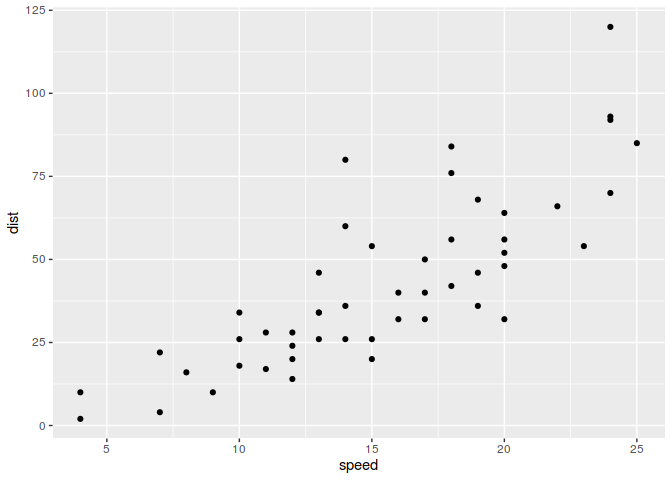{{ message }}
/ ggplot2 Public

Have a question about this project? Sign up for a free GitHub account to open an issue and contact its maintainers and the community.

By clicking “Sign up for GitHub”, you agree to our terms of service and privacy statement. We’ll occasionally send you account related emails.

# `NA` limits in coords#2907

Closed
opened this issue Sep 21, 2018 · 3 comments · Fixed by #3380
Closed

# `NA` limits in coords #2907

opened this issue Sep 21, 2018 · 3 comments · Fixed by #3380
Labels
coord 🗺️ feature a feature request or enhancement

### eliocamp commented Sep 21, 2018

Setting `NA` in limits of `coords` in a similar fashion to `scales` ends up in an error.

```library(ggplot2)

ggplot(cars, aes(speed, dist)) +
geom_point() +
coord_cartesian(ylim = c(25, NA))  # emulating scale_y_continuous(limits = c(25, NA))
#> Error in if (zero_range(range)) zero_width else diff(range): missing value where TRUE/FALSE needed```

Created on 2018-09-21 by the reprex package (v0.2.0).

I've tinker a bit and found that the error is in this line:

Line 121 in 01155ba

 range <- range(scale\$transform(limits))

`limits <- ifelse(is.na(limits), scale\$get_limits(), limits)`
solves the issue in this case.

```library(ggplot2)

scale_range <- function(scale, limits = NULL, expand = TRUE) {
expansion <- if (expand) ggplot2:::expand_default(scale) else c(0, 0)

if (is.null(limits)) {
scale\$dimension(expansion)
} else {
limits <- ifelse(is.na(limits), scale\$get_limits(), limits)
range <- range(scale\$transform(limits))
scales::expand_range(range, expansion, expansion)
}
}

assignInNamespace("scale_range", scale_range, ns = "ggplot2")

ggplot(cars, aes(speed, dist)) +
geom_point() +
coord_cartesian(ylim = c(25, NA))  ```Created on 2018-09-21 by the reprex package (v0.2.0).

I'm not versed enough on how `ggplot2` handles coords to know if it's a robust fix across different coords, though.

### atusy commented Nov 23, 2018

 There's another inconsistency among `coord_cartesian()` and `scale_*_continuous1()`. Limits for coord_cartesian can be length larger than 3 due to `range()` in `scale_range()` unlike limits for `scale_*_continuous()`. Thus, in `ifelse(is.na(limits), scale\$get_limits(), limits)` by @eliocamp, `scale\$get_limits()` will be re-cycled to have same length with `limits`. It may result in unexpected behavior. ```library(ggplot2) scale_range <- function(scale, limits = NULL, expand = TRUE) { expansion <- if (expand) ggplot2:::expand_default(scale) else c(0, 0) if (is.null(limits)) { scale\$dimension(expansion) } else { limits <- ifelse(is.na(limits), scale\$get_limits(), limits) range <- range(scale\$transform(limits)) scales::expand_range(range, expansion, expansion) } } assignInNamespace("scale_range", scale_range, ns = "ggplot2") ggplot(cars, aes(speed, dist)) + geom_point() + coord_cartesian(ylim = c(25, NA, NA))```Created on 2018-11-23 by the reprex package (v0.2.1) I think `limits` argument should only accept ``````- a finite value - a numeric vector of length 2 with or without NA `````` Is there any reason that `coord_cartesian` needs to accept `limits` with length larger than 2?

### hadley commented Jun 18, 2019

 I just tried to do this too!

This was referenced Jun 18, 2019

### lock bot commented Dec 28, 2019

 This old issue has been automatically locked. If you believe you have found a related problem, please file a new issue (with reprex) and link to this issue. https://reprex.tidyverse.org/

to subscribe to this conversation on GitHub. Already have an account? Sign in.
Labels
coord 🗺️ feature a feature request or enhancement
Projects
None yet
Development

Successfully merging a pull request may close this issue.